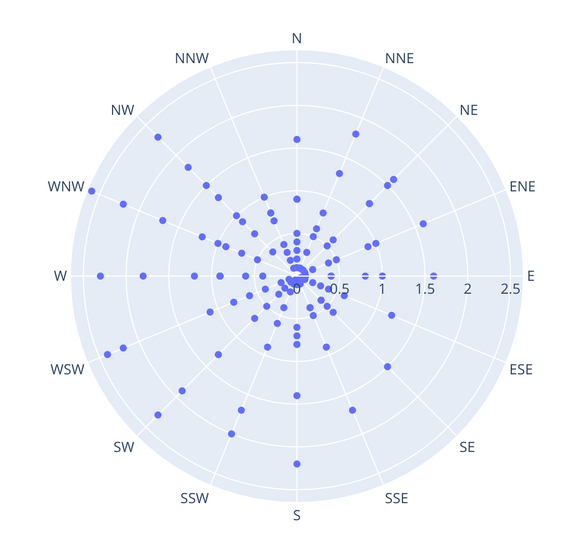# Polar scatter plots

Polar scatter plots are a chart type I regularly miss in Domo. Here's a picture of one grabbed randomly form the Plot.ly docs:https://plot.ly/python/polar-chart/

Polar scatter plots can help solve a couple of problems. Standard scatter plots are great, but tend to overwhelm the uninitiated. In fact, with a lot of data, or very skewed distributions, scatters can quickly turn into a jumble bunched up around 0,0. Two reasonable solutions are to either have an array of scatters (small multiples0 highlighting different categories side-by-side, all plotted to the same scale. Then you can quickly compare series. Domo doesn't seem to support small multiples, so that's out. Polar scatter plots are the other solution.

With a polar scatter, you've got 0,0 and the middle, and then the data radiates with two attributes, angle and distance. The angle is traditionally a compass point, but doesn't have to be. As an example, time series data can plot *really* well this way. The 360 degrees can be divided up by day of the week, hour of the day, day of the year, week of they year, etc. The distance/magnitude is whatever you're plotting. Color can be used for each category.

Fundamentally, you're plotting data that you could plot against time on the x and magnitude on the y. But, in a lot of cases, there are patterns an anomalies that pop out on a polar scatter that are obscured in a line or dot plot along a normal x timeline.

Domo has scatters, but only one-at-a-time. It also has radar (spider/Kiviat) diagrams, which are plotted on the same coordinate space as a polar scatter.

There are several variations on how to plot a polar chart, including wedges, etc. Of all of these, I've rarely found anything other than the scatter particularly useful. And, the best thing, it's *much* easier for people to grasp what they're seeing in a polar scatter plot than the same data in many other presentations.

It would be great if Domo added polar scatter plots, maybe in the Data Science theme? The math for these plots isn't hard, the existing radar chart, for example, is a harder plotting problem.

2